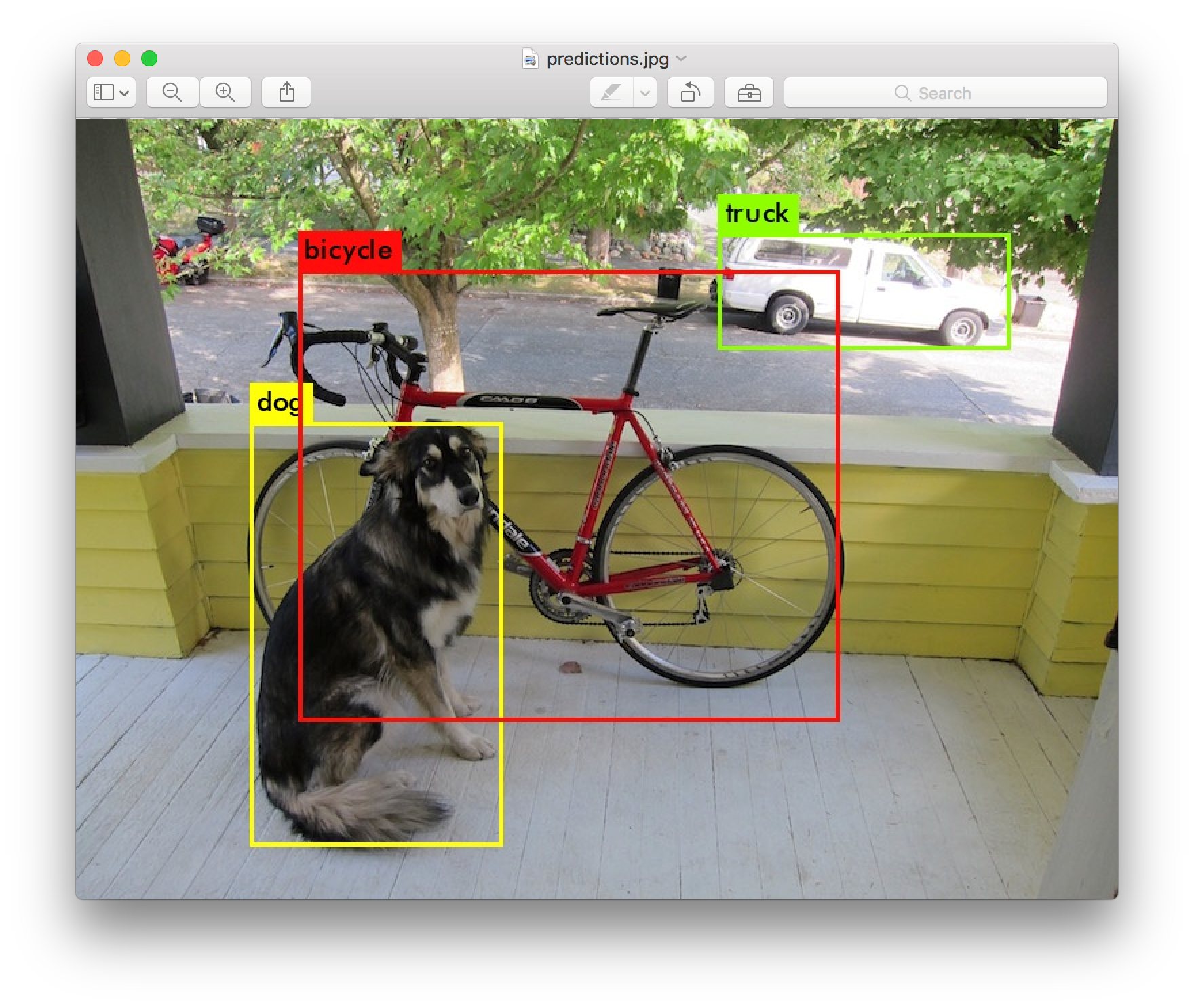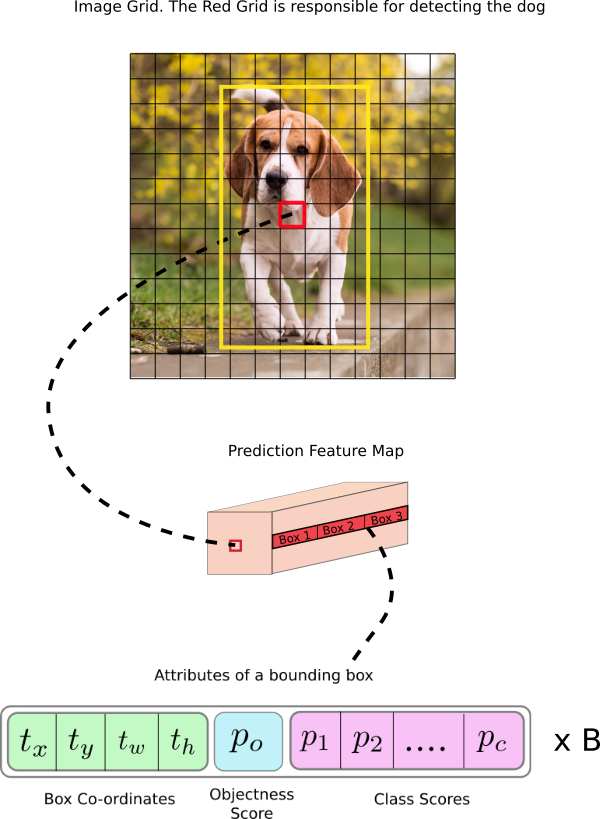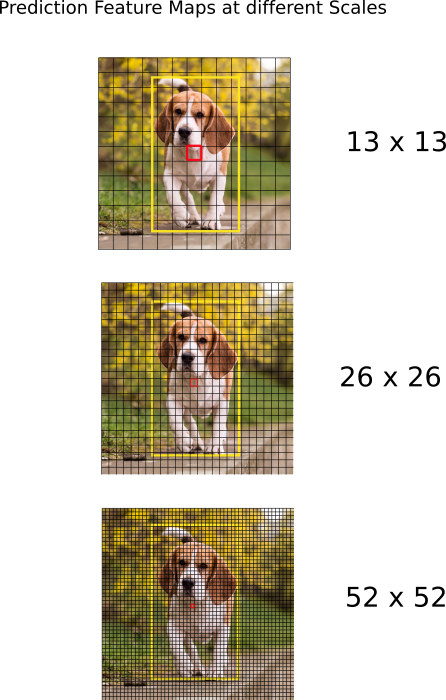×

Search anything:

# You only look once (YOLO) algorithm

#### Machine Learning (ML) object detection computer vision yoloYou only look once (YOLO) is a state-of-the-art, real-time object detection system. It is a fully convolutional network. On a Pascal Titan X, it processes images at 30 FPS and has a mAP of 57.9% on COCO test-dev.

### Comparision with other Detectors

YOLOv3 is extremely fast and accurate. In mAP measured at .5 IOU YOLOv3 is on par with Focal Loss but about 4x faster. Moreover, you can easily tradeoff between speed and accuracy simply by changing the size of the model, no retraining required!

### Performance on the COCO Dataset

Performance metrics on the COCO dataset are:

• mAP : 48.1
• FPS : 48

### How It Works?

Prior detection systems repurpose classifiers or localizers to perform detection. They apply the model to an image at multiple locations and scales. High scoring regions of the image are considered detections.

We use a totally different approach. We apply a single neural network to the full image. This network divides the image into regions and predicts bounding boxes and probabilities for each region. These bounding boxes are weighted by the predicted probabilities.

Our model has several advantages over classifier-based systems. It looks at the whole image at test time so its predictions are informed by global context in the image. It also makes predictions with a single network evaluation unlike systems like R-CNN which require thousands for a single image. This makes it extremely fast, more than 1000x faster than R-CNN and 100x faster than Fast R-CNN. See our paper for more details on the full system.### A Fully Convolution Neural Networks

YOLO makes use of only convolutional layers, making it a fully convolutional network (FCN). It has:

• 75 convolutional layers with skip connections and upsampling layers
• No form of pooling is used
• a convolutional layer with stride 2 is used to downsample the feature maps. This helps in preventing loss of low-level features often attributed to pooling.

Being a FCN, YOLO is invariant to the size of the input image. However, in practice, we might want to stick to a constant input size due to various problems that only show their heads when we are implementing the algorithm.

A big one amongst these problems is that if we want to process our images in batches (images in batches can be processed in parallel by the GPU, leading to speed boosts), we need to have all images of fixed height and width. This is needed to concatenate multiple images into a large batch.The network downsamples the image by a factor called the stride of the network. For example, if the stride of the network is 32, then an input image of size 416 x 416 will yield an output of size 13 x 13. Generally, stride of any layer in the network is equal to the factor by which the output of the layer is smaller than the input image to the network.

### Interpreting the output

Typically, (as is the case for all object detectors) the features learned by the convolutional layers are passed onto a classifier/regressor which makes the detection prediction (coordinates of the bounding boxes, the class label.. etc).

In YOLO, the prediction is done by using a convolutional layer which uses 1 x 1 convolutions.

Now, the first thing to notice is our output is a feature map.

Since we have used 1 x 1 convolutions, the size of the prediction map is exactly the size of the feature map before it. In YOLO v3 (and it's descendants), the way you interpret this prediction map is that each cell can predict a fixed number of bounding boxes.Depth-wise, we have (B x (5 + C)) entries in the feature map. B represents the number of bounding boxes each cell can predict. According to the paper, each of these B bounding boxes may specialize in detecting a certain kind of object. Each of the bounding boxes have 5 + C attributes, which describe the center coordinates, the dimensions, the objectness score and C class confidences for each bounding box. YOLO v3 predicts 3 bounding boxes for every cell.

You expect each cell of the feature map to predict an object through one of it's bounding boxes if the center of the object falls in the receptive field of that cell.### Implementation

YOLO can only detect objects belonging to the classes present in the dataset used to train the network. We will be using the official weight file for our detector. These weights have been obtained by training the network on COCO dataset, and therefore we can detect 80 object categories.

That's it for the first part. This post explains enough about the YOLO algorithm to enable you to implement the detector. However, if you want to dig deep into how YOLO works, how it's trained and how it performs compared to other detectors, you can read the original papers, the links of which I've provided below.